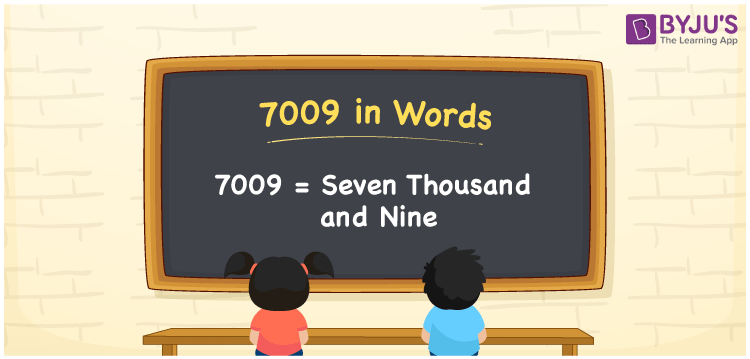# 7009 in words

7009 in words is written as Seven Thousand and Nine. 7009 represents the count or value. The article on Counting Numbers can give you an idea about count or counting. The number 7009 is used in expressions that relate to money, distance, length, year and others. Let us consider an example for 7009. ”I could record only Seven Thousand and Nine steps yesterday.”

 7009 in words Seven Thousand and Nine Seven Thousand and Nine in Numbers 7009

## 7009 in English Words## How to Write 7009 in Words?

We can convert 7009 to words using a place value chart. The number 7009 has 4 digits, so let’s make a chart that shows the place value up to 4 digits.

 Thousands Hundreds Tens Ones 7 0 0 9

Thus, we can write the expanded form as:

7 × Thousand + 0 × Hundred + 0 × Ten + 9 × One

= 7 × 1000 + 0 × 100 + 0 × 10 + 9 × 1

= 7009

= Seven Thousand and Nine.

7009 is the natural number that is succeeded by 7008 and preceded by 7010.

7009 in words – Seven Thousand and Nine.

Is 7009 an odd number? – Yes.

Is 7009 an even number? – No.

Is 7009 a perfect square number? – No.

Is 7009 a perfect cube number? – No.

Is 7009 a prime number? – No.

Is 7009 a composite number? – Yes.

## Solved Example

1. Write the number 7009 in expanded form

Solution: 7 × 1000 + 0 × 100 + 0 × 10 + 9 × 1

We can write 7009 = 7000 + 000 + 00 + 9

= 7 × 1000 + 0 × 100 + 0 × 10 + 9 × 1

## Frequently Asked Questions on 7009 in words

Q1

### How to write 7009 in words?

7009 in words is written as Seven Thousand and Nine.
Q2

### Is 7009 a perfect square number?

No. 7009 is not a perfect square number.
Q3

### Is 7009 a prime number?

No. 7009 is not a prime number.